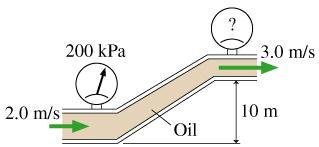# Problem: What does the top pressure gauge in the figure read?

###### FREE Expert Solution

We are required to use Bernoulli's equation to determine the pressure at the outlet.

Bernoulli's equation:

$\overline{){{\mathbf{P}}}_{{\mathbf{1}}}{\mathbf{+}}\frac{\mathbf{1}}{\mathbf{2}}{\mathbf{\rho }}{{{\mathbf{v}}}_{{\mathbf{1}}}}^{{\mathbf{2}}}{\mathbf{+}}{{\mathbf{h}}}_{{\mathbf{1}}}{\mathbf{\rho }}{\mathbf{g}}{\mathbf{=}}{{\mathbf{P}}}_{{\mathbf{2}}}{\mathbf{+}}\frac{\mathbf{1}}{\mathbf{2}}{\mathbf{\rho }}{{\mathbf{v}}_{\mathbf{2}}}^{{\mathbf{2}}}{\mathbf{+}}{{\mathbf{h}}}_{{\mathbf{2}}}{\mathbf{\rho }}{\mathbf{g}}}$

99% (195 ratings)###### Problem DetailsWhat does the top pressure gauge in the figure read?